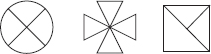# User ForumSubject :IMO    Class : Class 2

Pinki has some stickers.There are _____ triangles in the stickers altogether.

A 6
B 8
C 10
D 12

Class : Class 3
ans is b

Class : Class 4

## Ans 3:

Class : Class 4
Answer is option (B), 8THANKS. :D

Class : Class 2

Class : Class 2
a,b,c,d

Class : Class 4
D

## Ans 7:

Class : Class 4
Answer is option (B), because 1st Step - we cannot count the first figure triangles because these are mentioned under the (curved) or (circle) shaped. 2nd Step - In the second figure , there are 4 triangles which we can count as it is not like as metioned as first figure..... 3rd Step - In the third figure we can only see 3 triangles but then also you guys tought that answer is d but this is not the correct way! because can you all se the 2 triangles? Ok and the triangle in which the 2 triangles are mentioned is the large triangle and the correct sum is 4 4 = 8 triangles. Hence the answer is option (B). Girls are best than boys "O"!!

Class : Class 8
B

## Ans 9:

Class : Class 4
4 3 = 7 not eight

## Ans 10:

Class : Class 3
the first stikcer (circle) also has some triangles....

Class : Class 4

## Ans 12:

Class : Class 3
the correct answer is wrong ... there are 12 triangles ....so, the correct answer is D.

## Ans 13:

Class : Class 2
the first one is 4 triangles then there are 4 triangles again so 4+4=8 then plus 3 more 8+3=11 but the answer is not there so you can choose anything near to 11 wichs is12 so hence the answer is option d

Class : Class 3